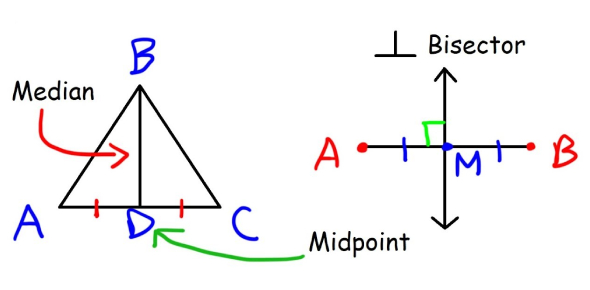# Geometry Knowledge Trivia Exam: Quiz!

13 Questions | Total Attempts: 505SettingsGeometry is the division of mathematics that employs measurement, properties, and relationships of points, lines, angles, surfaces, and solids, and figures in space from their defining conditions. As it relates to this quiz, you should be aware of which type of statement is always false, what biconditional means, what is true about vertical angles, and what happens when two lines are perpendicular. This quiz will measure your knowledge of geometry; give it a try.

• 1.
A hypothesis is ALWAYS at the beginning of the sentence, and the conclusion is ALWAYS at the end.
• A.

True

• B.

False

• 2.
Which type of statement is always false?
• A.

Hypothesis

• B.

Conclusion

• C.

Counterexample

• D.

Converse

• 3.
Biconditional must contain the linking phrase:
• A.

If and only if

• B.

Then

• C.

Implies

• D.

Only if

• 4.
Vertical angles....
• A.

Are never congruent

• B.

Are always congruent

• C.

Are sometimes congruent

• D.

Are not in the same picture

• E.

Don't exist.

• 5.
Complementary angles...
• A.

Complement each other.

• B.

Are two angles whose sum is 90.

• C.

Are two angles whose sum is 180.

• D.

Are two angles that are congruent.

• E.

Are two angles that are less than 45 degrees.

• 6.
Supplementary angles...
• A.

Angles that mean the opposite of complementary.

• B.

Two angles whose sum is 90.

• C.

Two angles whose sum is 180.

• D.

Two angles that are congruent.

• E.

Two angles that are less than 45 degrees.

• 7.
What is the equation for a complementary angle?
• A.

X=x

• B.

90-x=

• C.

180-x=

• D.

X-90=

• E.

X-180=

• 8.
What is the equation for a supplementary angle?
• A.

X=x

• B.

90-x=

• C.

180-x=

• D.

X-90=

• E.

X-180=

• 9.
If two lines are perpendicular...
• A.

Then they form congruent adjacent angles

• B.

They don't intersect.

• C.

Then the angles are complementary

• D.

They extend forever.

• 10.
If two lines form congruent adjacent angles...
• A.

The angles are supplementary

• B.

Then the angles are complementary

• C.

They don't intersect.

• D.

Then the lines are perpendicular.

• 11.
If two angles are supplements of congruent angles...
• A.

They equal 180

• B.

They equal 90

• C.

Then the two angles are congruent.

• D.

They're angles are always vertical.

• E.

They've been conquered by zombies.

• 12.
If two angles are complements of congruent angles...
• A.

Then the two angles are congruent.

• B.

They equal 180

• C.

They equal 90

• D.

Then their angles are obtuse.

• E.

The zombies killed all the humans.

• 13.
What is the Midpoint Theorem?
• A.

When the definition expresses that a segment is half of the segment its in.

• B.

When there is a dot at the midpoint.

• C.

A dumb theorem.

• D.

Whats a theorem?

Related TopicsBack to top